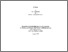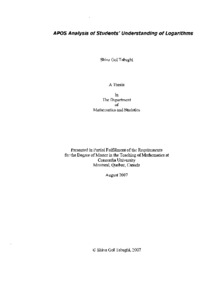Title:

# APOS analysis of students' understanding of logarithms

Gol Tabaghi, Shiva (2007) APOS analysis of students' understanding of logarithms. Masters thesis, Concordia University.Preview Text (application/pdf) MR34693.pdf - Accepted Version 6MB

## Abstract

This research is aimed at analyzing students' understanding of the concept of logarithms. Traditionally students are introduced to logarithmic functions as the inverse of exponential functions, even though logarithms were invented independently of exponents. In this study, a review of the historical genesis of logarithms, the literature review on the understanding of exponential and logarithmic functions, and a review of several textbooks from different times and geographical places are presented. These reviews allow the identification of two notions of logarithms: arithmetic and functional. Even though current curriculum introduces the functional notion of logarithms, attained curriculum focuses on the arithmetic notion of logarithms. In addition, the importance of logarithms, that is, their use in converting multiplication of numbers into addition of logarithms of numbers, has not been highlighted. I conducted six clinical interviews with students from prerequisite mathematics courses and a core mathematics course. This data is analyzed within the context of APOS theory to identify students' difficulties in understanding the concept of logarithms. The results reveal that most students' understanding of the arithmetic notion of logarithms does not go beyond the "process" level, since understanding the arithmetic notion of logarithms as undoing what exponentiation does require an understanding of exponentiation with real exponents.

Divisions: Concordia University > Faculty of Arts and Science > Mathematics and Statistics Thesis (Masters) Gol Tabaghi, Shiva viii, 166 leaves ; 29 cm. Concordia University M.T.M. Teaching of Mathematics 2007 Sierpinska, Anna LE 3 C66M38M 2007 G65 975433 Concordia University Library 22 Jan 2013 16:08 13 Jul 2020 20:07 https://concordiauniversity.on.worldcat....
All items in Spectrum are protected by copyright, with all rights reserved. The use of items is governed by Spectrum's terms of access.

Repository Staff Only: item control pageResearch related to the current document (at the CORE website)
Back to top